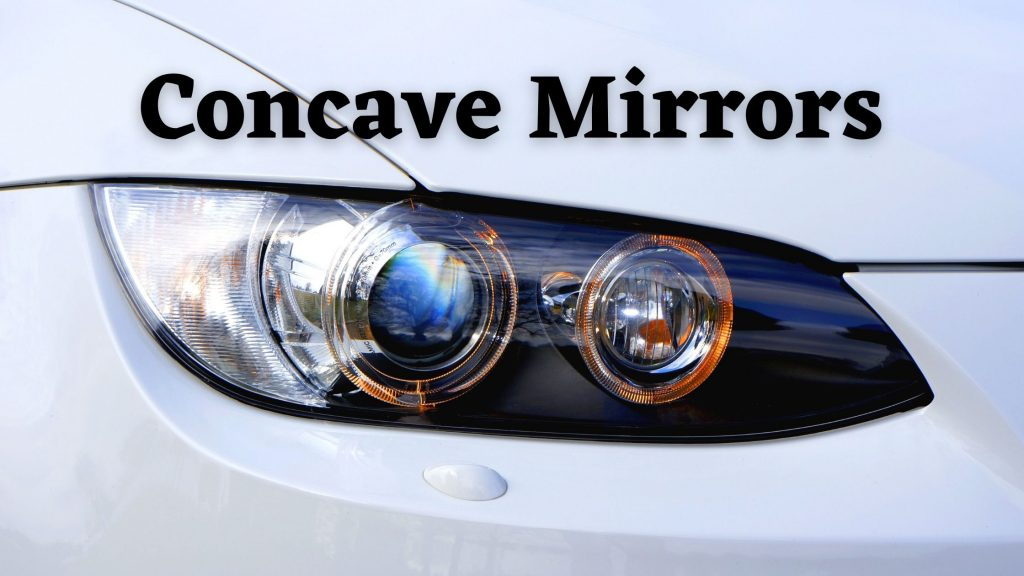Physics
Study Material

# Concave Mirror- Principal Focus, Image Formation, Uses

A concave mirror has a reflective surface that is curved inward and away from the light source. Concave mirrors reflect light inward to one focal point.

3 minutes long
Posted by Mahak Jain, 28/7/2021Hesap Oluştur

Got stuck on homework? Get your step-by-step solutions from real tutors in minutes! 24/7. Unlimited.

Spherical mirrors and lenses have made a very important and integral part of life. Many of us might be visionless without these mirrors and lenses. Concave and convex mirrors are most commonly used spherical mirrors .Learn about concave mirror in this article.

## Define Concave Mirror

If a hollow sphere is cut into parts and the outer surface of the cut part is silvered, then it becomes a mirror with its inner side as the reflecting surface. This type of mirror is known as a concave mirror.

## Sign Convention for Concave Mirrors

Directions taken are as follows and shown in figure below:

• Distances behind silvered face of mirror are positive.
• Distances in front of reflecting face are negative.
• Height above principal axis is positive.
• Height below principal axis is negative.

## Define The Principal Focus of a Concave Mirror

Light rays that are parallel to the principal axis converge at a specific point on its principal axis after reflecting from the mirror. This point the principal focus of the concave mirror. Point F is principal focus in the image below.

Note that, focal length for concave mirrors are negative.

## Radius of Curvature and Focal Length of Concave Mirror

Radius of the sphere of which mirror is a part is the radius of curvature. In simple concave mirrors (covered in this blog) focal length is half of radius of curvature.

Thus, f = c/2

## Characteristics of Concave Mirror

• Light converges at a point when it strikes and reflects back from the reflecting surface of the concave mirrors. Hence, the name converging mirror.
• When we place the concave mirror very close to the object, we obtain a magnified and virtual image.
• The image formed by the concave mirrors can be small or large or can be real or virtual.

## Uses of Concave Mirror

### Common Uses

Concave mirrors is used in

• Searchlights
• Shaving mirrors
• Satellite dishes
• Reflectors in torches and headlights

Uses of Concave Mirrors in Science.

• Used in microscopes as condensers.
• Reflecting telescopes have concave mirrors to collect light from distant sources.